# ML Aggarwal Solutions for Class 7 Maths Chapter 1 Integers

ML Aggarwal Solutions for Class 7 Maths Chapter 1 Integers, are given here in a simple but comprehensive way. The Integers solutions are extremely helpful for students to clear all their doubts easily and understand the basics of the Chapter more effectively. It is essential to understand the various kinds of questions and figure out the best answers for them. Students find ML Aggarwal Solutions for Class 7 Maths Chapter 1 in downloadable PDF format.

Chapter 1 – Integers are whole numbers that can be positive or negative or can be zero. In this ML Aggarwal Solutions for Class 7 Maths Chapter 1 students are going to study more about Integers, their properties and operations. Likewise, the addition and the subtraction of the integers, properties of addition and subtraction of integers, multiplication and division of integers, properties of multiplication and division of integers, representation of integers on number line.

## Download the PDF of ML Aggarwal Solutions for Class 7 Maths Chapter 1 Integers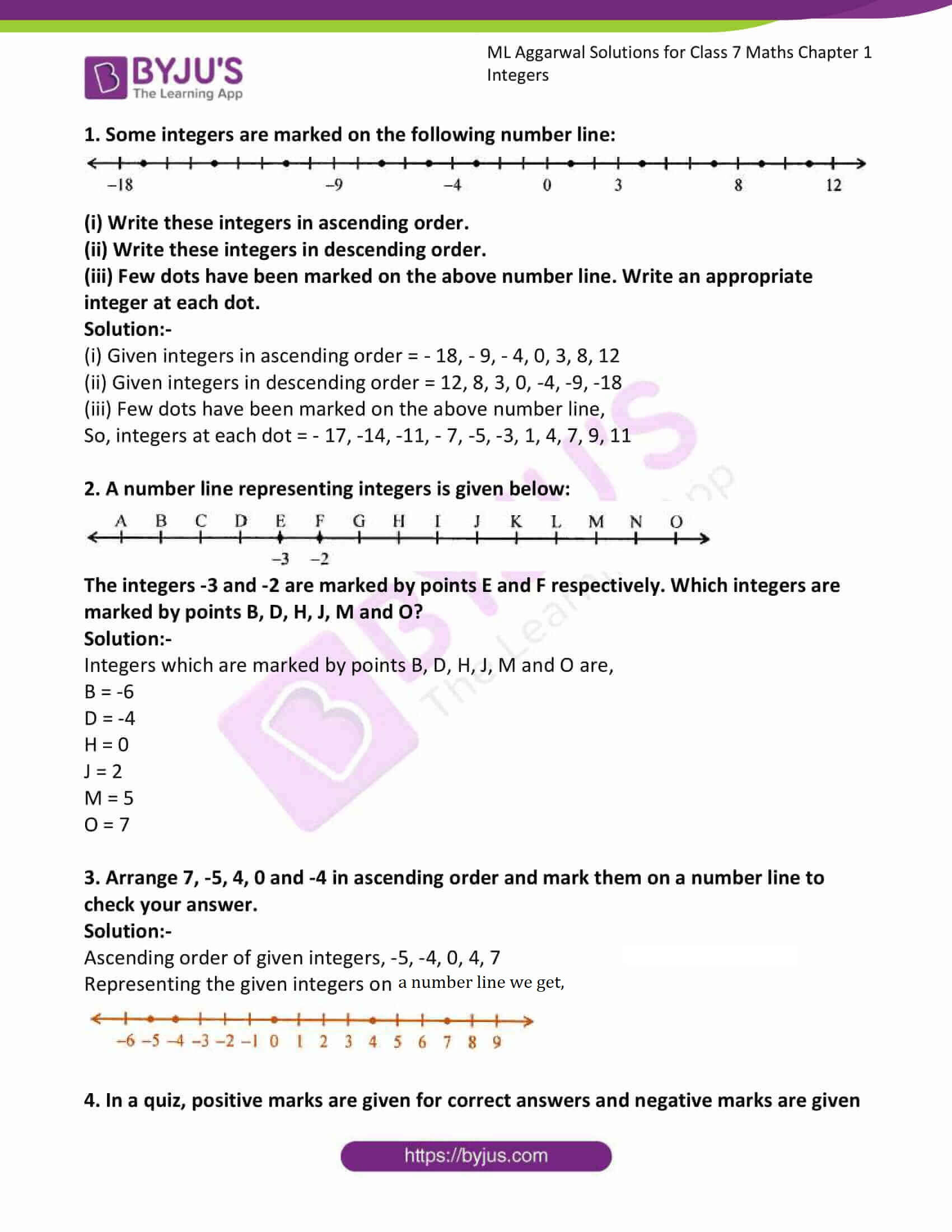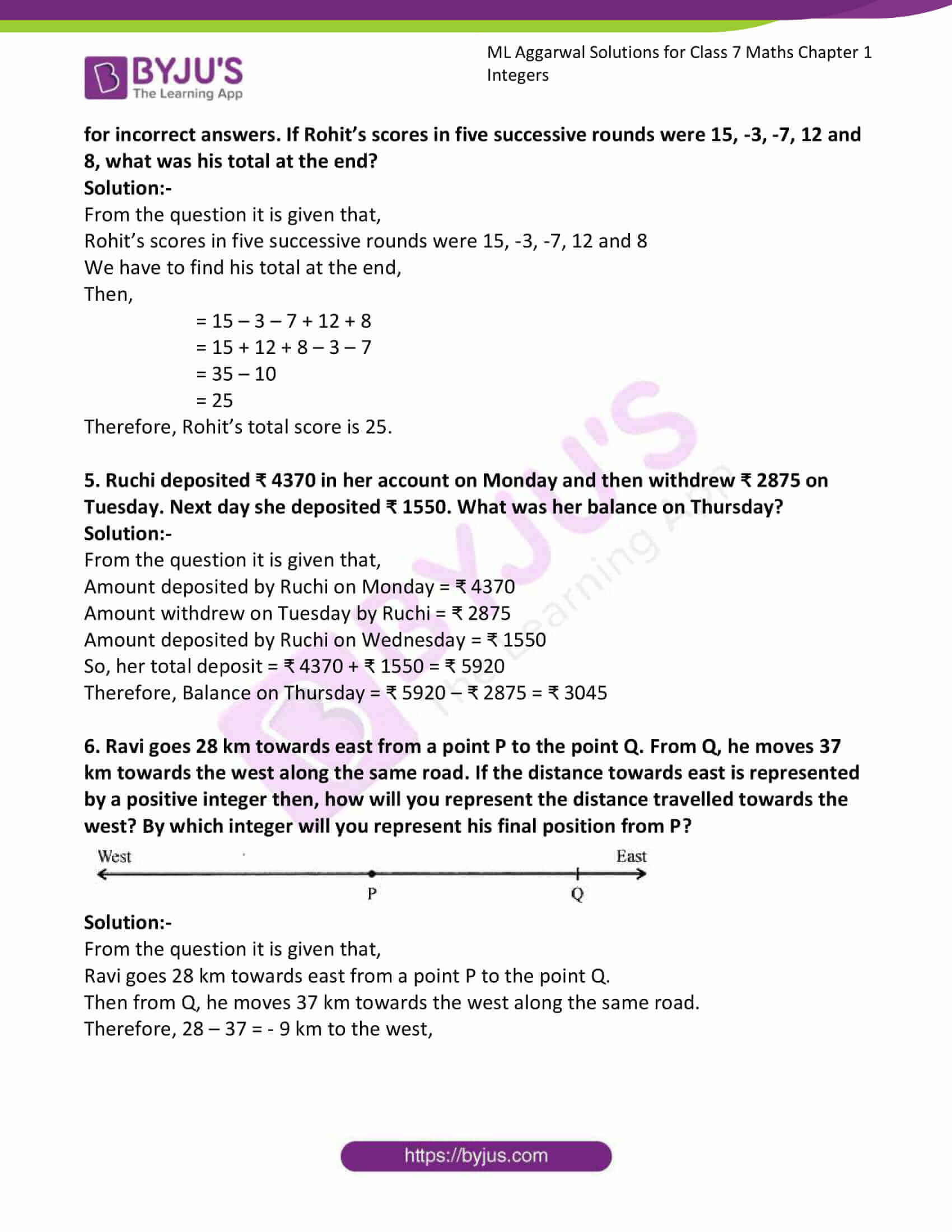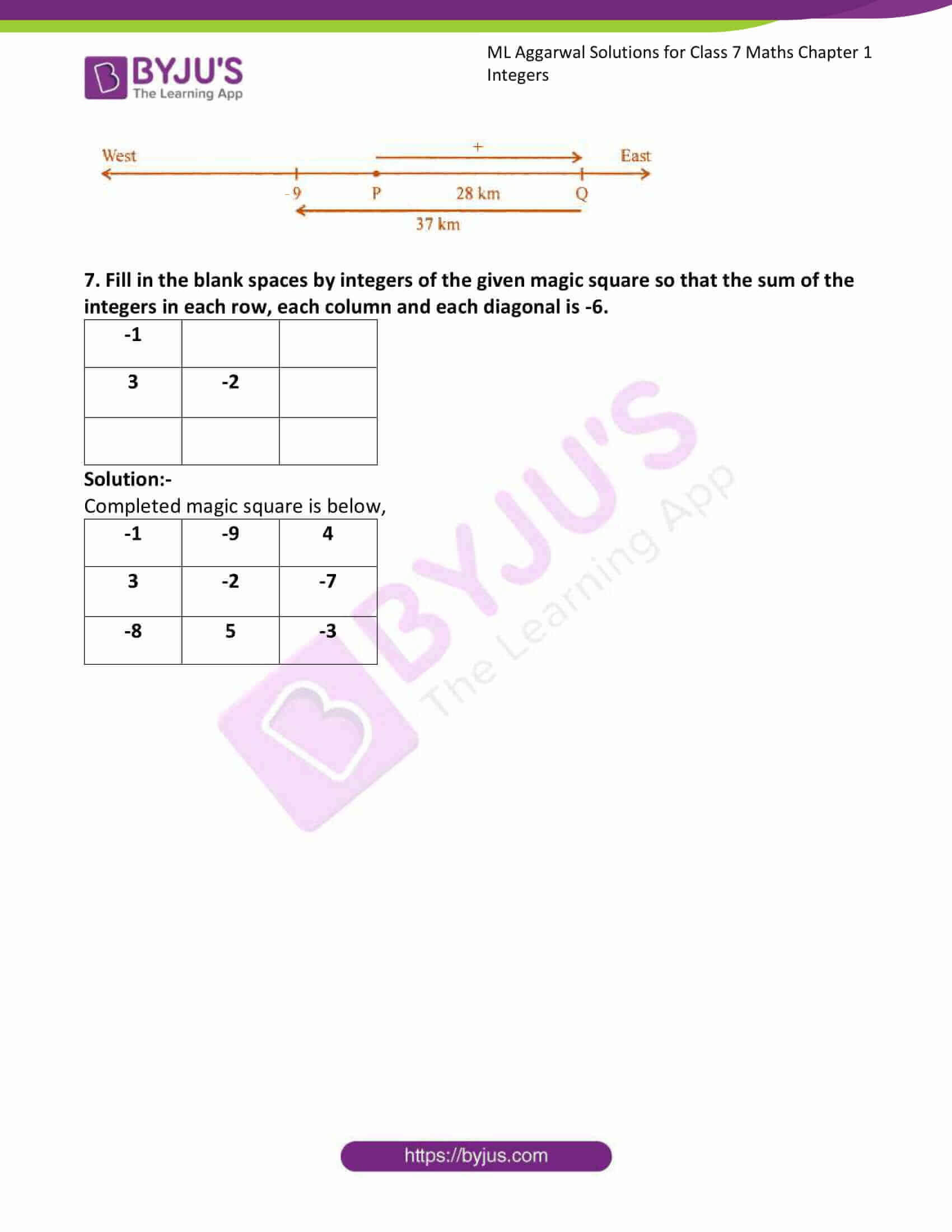### Access answers to ML Aggarwal Solutions for Class 7 Maths Chapter 1 Integers

1. Some integers are marked on the following number line: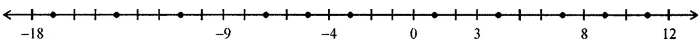(i) Write these integers in ascending order.
(ii) Write these integers in descending order.
(iii) Few dots have been marked on the above number line. Write an appropriate integer at each dot.

Solution:-

(i) Given integers in ascending order = – 18, – 9, – 4, 0, 3, 8, 12

(ii) Given integers in descending order = 12, 8, 3, 0, -4, -9, -18

(iii) Few dots have been marked on the above number line,

So, integers at each dot = – 17, -14, -11, – 7, -5, -3, 1, 4, 7, 9, 11

2. A number line representing integers is given below: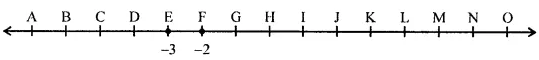The integers -3 and -2 are marked by points E and F respectively. Which integers are marked by points B, D, H, J, M and O?

Solution:-

Integers which are marked by points B, D, H, J, M and O are,

B = -6

D = -4

H = 0

J = 2

M = 5

O = 7

3. Arrange 7, -5, 4, 0 and -4 in ascending order and mark them on a number line to check your answer.
Solution:-

Ascending order of given integers, -5, -4, 0, 4, 7

Representing the given integers on a number line we get,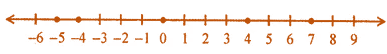4. In a quiz, positive marks are given for correct answers and negative marks are given for incorrect answers. If Rohit’s scores in five successive rounds were 15, -3, -7, 12 and 8, what was his total at the end?
Solution:-

From the question it is given that,

Rohit’s scores in five successive rounds were 15, -3, -7, 12 and 8

We have to find his total at the end,

Then,

= 15 – 3 – 7 + 12 + 8

= 15 + 12 + 8 – 3 – 7

= 35 – 10

= 25

Therefore, Rohit’s total score is 25.

5. Ruchi deposited ₹ 4370 in her account on Monday and then withdrew ₹ 2875 on Tuesday. Next day she deposited ₹ 1550. What was her balance on Thursday?
Solution:-

From the question it is given that,

Amount deposited by Ruchi on Monday = ₹ 4370

Amount withdrew on Tuesday by Ruchi = ₹ 2875

Amount deposited by Ruchi on Wednesday = ₹ 1550

So, her total deposit = ₹ 4370 + ₹ 1550 = ₹ 5920

Therefore, Balance on Thursday = ₹ 5920 – ₹ 2875 = ₹ 3045

6. Ravi goes 28 km towards east from a point P to the point Q. From Q, he moves 37 km towards the west along the same road. If the distance towards east is represented by a positive integer then, how will you represent the distance travelled towards the west? By which integer will you represent his final position from P?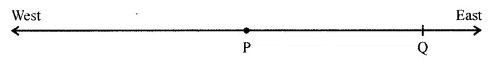Solution:-

From the question it is given that,

Ravi goes 28 km towards east from a point P to the point Q.

Then from Q, he moves 37 km towards the west along the same road.

Therefore, 28 – 37 = – 9 km to the west,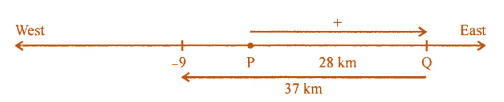7. Fill in the blank spaces by integers of the given magic square so that the sum of the integers in each row, each column and each diagonal is -6.

 -1 3 -2

Solution:-

Completed magic square is below,

 -1 -9 4 3 -2 -7 -8 5 -3